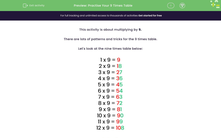# Practise Your 9 Times Table

In this worksheet, students will learn to multiply by 9.Key stage:  KS 2

Curriculum topic:   Number: Multiplication and Division

Curriculum subtopic:   Know Multiplication Tables up to 12 x 12

Difficulty level:#### Worksheet Overview

This activity is about multiplying by 9.

There are lots of patterns and tricks for the 9 times table.

Let's look at the nine times table below:Pattern 1:
From 2 × 9 onwards, the tens digit counts up and the ones digit counts down. For 12 × 9 we have 10 tens, which is 100.

Pattern 2:
Each product of the nine times table has an eventual digit sum of 9. This means that we can check if our answer is likely to be correct.

For example:

9 × 9 = 81 and 8 + 1 = 9

11 × 9 = 99 and 9 + 9 = 18 then 1 + 8 = 9.

Pattern 3:
An easy way to multiply by 9 is to multiply by 10 and then count back one lot.

For example: 5 × 9

Step 1: 5 × 10 = 50

Step 2: 50 - 5 = 45

5 × 9 = 45Let's try an example question together!

Example

A cookie has 9 chocolate chips.

How many chocolate chips do 6 cookies have?

What is 9 times 6?

Using our pattern, we know that nine lots of six is fifty-four.

9 × 6 = 54

Now try the questions for yourself.

### What is EdPlace?

We're your National Curriculum aligned online education content provider helping each child succeed in English, maths and science from year 1 to GCSE. With an EdPlace account you’ll be able to track and measure progress, helping each child achieve their best. We build confidence and attainment by personalising each child’s learning at a level that suits them.

Get started••••